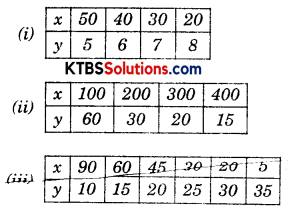# KSEEB Solutions for Class 8 Maths Chapter 13 Direct and Inverse Proportions InText Questions

## KSEEB Solutions for Class 8 Maths Chapter 13 Direct and Inverse Proportions InText Questions

Try These (Page 211)

Question 1.
Observe the following tables and find which pair of variables (here x and y) are in inverse proportion.Solution:
Two variables are inversly proportional of product of both is constant.
(i) x × y = 50 × 5 = 250
= 40 × 6 = 240
= 30 × 7 = 210
= 20 × 8 = 160
∴ 250 ≠ 240 ≠ 210 ≠ 160
Hence not inversly proportional

(ii) x × y = 100 × 60 = 6000
= 200 × 30 = 6000
= 300 × 20 = 6000
= 400 × 15 = 6000
all are equal.
Hence inversely proportional.(iii) x × y = 90 × 10 = 900
= 60 × 15 = 900
= 45 × 20 = 900
= 30 × 25 = 750
= 20 × 30 = 600
= 5 × 35 = 175
900 ≠ 750 ≠ 600 ≠ 175
Hence x and y are not inversely proportional.